# Chapter 11 Area Related to Circles NCERT Exemplar Solutions Exercise 11.4 Class 10 Maths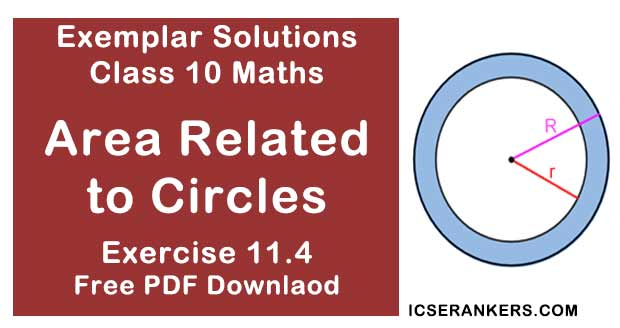Chapter Name NCERT Maths Exemplar Solutions for Chapter 11 Area Related to Circles Exercise 11.4 Book Name NCERT Exemplar for Class 10 Maths Other Exercises Exercise 11.1Exercise 11.2Exercise 11.3 Related Study NCERT Solutions for Class 10 Maths

### Exercise 11.4 Solutions

1. The area of a circular playground is 22176 m2 . Find the cost of fencing this ground at the rate of Rs 50 per metre.

Solution
Area of the circular playground = 22176 m2
Let r be the radius of the circle.
πr2 = 22176
⇒ (22/7)r2 = 22176
⇒ r2 = 22176 × (22/7)
⇒ r2 = 7056
⇒ r = 84
Radius of the circular playground = 84 m
Circumference of the circle = 2πr
= 2 × (22/7) × 84
= 528 m
Cost of fencing 1 meter of ground = Rs. 50
Cost of fencing the total ground = Rs. 528 × 50
= Rs. 26,400

2. The diameters of front and rear wheels of a tractor are 80 cm and 2 m respectively. Find the number of revolutions that rear wheel will make in covering a distance in which the front wheel makes 1400 revolutions.

Solution

Diameter of front wheels = d1  = 80 cm
Diameter of rear wheels = d2 = 2 m = 200 cm
Let r1 be the radius of the front wheels = 80/2 = 40 cm
Let r2 be the radius of the rear wheels = 200/2 = 100 cm
Circumference of the front wheels = 2πr
= 2 × (22/7) × 40
= 1760/7   cm
Circumference of the rear wheels = 2πr
= 2 × (22/7) × 100
= 4400/7 cm
No. of revolutions made by the front wheel = 1400
∴ Distance covered by the front wheel  = 1400 × (1760/7)
= 352000 cm
Number of revolutions made by rear wheel in covering a distance in which the front wheel makes 1400 revolutions,
= (Distance covered by front wheel)/(Circumference of rear wheel)
= 352000/4400/7
= (352000 × 7)/4400
= 560 revolutions.

3. Sides of a triangular field are  15m, 16m and 17m. With the three corners of the field a cow, a buffalo and a horse are tied separately with ropes of length 7 m each to graze in the field. Find the area of the field which cannot be grazed by the three animals.

Solution

Sides of the triangle are 15m, 16m, and 17m.
Now,
Perimeter of the triangle  = (15 + 16 + 17)m
= 48 m
Semi - perimeter of the triangle = s
= 48/2
= 24 m
By Heron's formula,
Area of the triangle =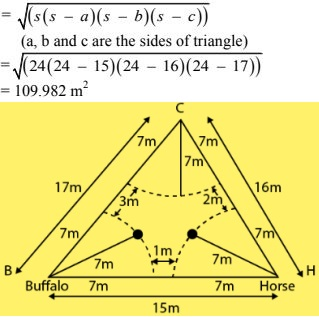Let B, C and H be the corners of the triangle on which buffalo, cow and horse are tied respectively with ropes of 7 m each.

So, the area grazed by each animal will be in the form of a sector.
Radius of each sector = r
= 7 m
Let x, y, z be the angles at corners B, C, H respectively.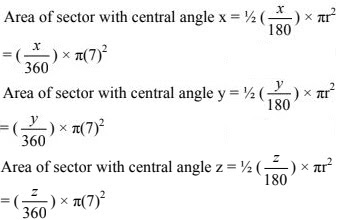Area of field not grazed by the animals = Area of triangle – (area of the three sectors)
= 109.892 – 77
= 32.982 cm2

4. Find the area of the segment of a circle of radius 12 cm whose Corresponding sector has a central angle of 60° (Use π = 3.14).

Solution

Radius of the circle = r
= 12 cm
OA = OB = 12 cm
∠ AOB = 60°  (given)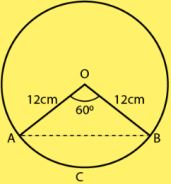As triangle OAB is an isosceles triangle,
∠ OAB = ∠ OBA = θ   (let)
And, Sum of interior angles of a triangle is 180°,
θ + θ + 60° = 180°
⇒ 2θ = 120°
⇒ θ = 60°
Therefore, the triangle AOB is an equilateral triangle.
AB = OA = OB = 12 cm
Area of the triangle AOB = (√3/4) × a2
where a is the side of the triangle.
= (√3/4) × (12)2
= (√3/4) × 144
= 36√3 cm2
= 62.354 cm2
Also, Central angle of the sector AOBCA = ∅
= 60°
= 60π/180
So, area of the sector AOBCA = (1/2)r2 ∅
= (1/2) × 122 × π/3
= 75.36 cm2
Area of the segment ABCA  = Area of the sector AOBCA - Area of the triangle AOB
= (75.36 - 62.354)cm2
= 13.006 cm2

5. A circular pond is 17.5 m is of diameter. It is surrounded by a 2 m wide path. Find the cost of constructing the path at the rate of Rs. 25 per m2 .

Solution

Diameter of the circular pond = 17.5 m
Let r be the radius of the park = r
r = 8.75 m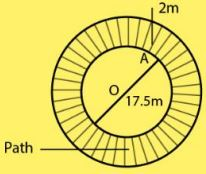The circular pond is surrounded by a path of width 2 m.
Radius of the outer circle = R
= (8.75+2) m
= 10.75 m
Area of the road = Area of the outer circular path – Area of the circular pond
πr2 - πR2
= 3.14 × (10.75)2 - 3.14 × (8.75)2
= 3.14 × (10.75)2 - (8.75)2
= 3.14 × (10.75 + 8.75) × 10.75 - 8.75)
= 3.14 × 19.5 × 2
= 122. 46 m2
So, the area of the path is 122.46 m2 .
Now, Cost of constructing the path per m2 = Rs. 25
Cost of constructing 122.46 m2 of the path  = Rs. 25 × 122.46
= Rs. 3061.50

6. In Fig., ABCD is a trapezium with AB || DC, AB = 18 cm, DC = 32 cm and distance between AB and DC = 14 cm. If arcs of equal radii 7 cm with centers A, B, C and D have been drawn, then find the area of the shaded region of the figure.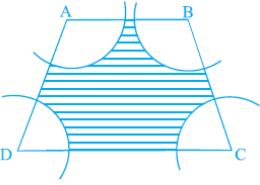Solution

AB = 18 cm,
DC = 32 cm
Distance between AB and DC = Height = 14 cm
Now,
Area of the trapezium = (1/2) × (Sum of parallel sides) × Height
= (1⁄2) × (18+32) × 14
= 350 cm2
We have,
AB ∥ DC,
∠ A +∠ D = 180°
∠ B +∠ C = 180°
Radius of each arc = 7 cm
Area of sector with ∠A = (∠A/180) × πr2
Area of sector with ∠B = (∠B/360) × πr2
Area of sector with ∠C = (∠C/360) × πr2
Area of sector with ∠D = (∠D/360) × πr2
Therefore,
Sum of total areas of sectors =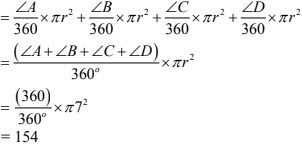Area of shaded region = Area of trapezium - (Total area of sectors)
= 350 - 154
= 196 cm2
Therefore, the required area of shaded region is 196 cm2.

7. Three circles each of radius 3.5 cm are draw in such a way that each of them touches the other two. Find the area enclosed between these circles.

Solution

The three circles are drawn such that each of them touches the other two.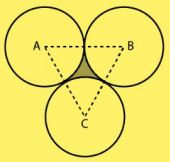By joining the centers of the three circles,
AB = BC = CA = 2(radius)
= 7 cm
So, triangle ABC is an equilateral triangle with each side 7 cm.
Area of the triangle = (√3/4) × a2 , Where a is the side of the triangle.
= (√3/4) × 72
= (49√3/4)  cm2
= 21.2176 cm2
Central angle of each sector = 60°
= (60π/180)
Area of each sector = (1/2)r2 θ
= (1/2) × (3.5)2 × (π/3)
= 12.25 × [22/(7×6)]
= 6.4167 cm2
Total area of three sectors = 3 × 6.4167
= 19.25 cm2
Area enclosed between three circles = Area of triangle ABC - Area of the three sectors
= 21.2176 - 19.25
= 1.9676 cm2
Therefore, the required are enclosed between these circles is 1.967 cm2 (approx).

8. Find the area of the sector of a circle of radius 5 cm, if the corresponding arc length is 3.5 cm.

Solution

Radius of the circle  = r = 5 cm
Arc length of the sector = l = 3.5 cm
Let the central angle (in radians ) be θ.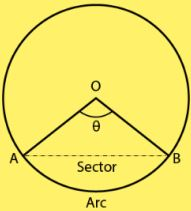We have,
Central angle (θ) = Arc length / Radius
= l/r
= 3.5/5
Area of the sector = (1⁄2) × r2θ
= (1⁄2) × 25 × 0.7
= 8.75 cm2
Therefore, required area of the sector of a circle is 8.75 cm2.

9. Four circular cardboard pieces of radii 7 cm are placed on a paper in such a way that each piece touches other two pieces. Find the area of the portion enclosed between these pieces.

Solution

The four circles are placed such that each piece touches the other two pieces.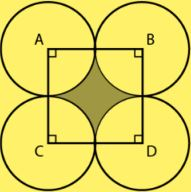By joining the centers of the circles by a line segment, we get a square ABDC with sides,
AB = BD = DC = CA = 2(Radius)
= 2(7) cm
= 14 cm
Area of the square = (Side)2
= (14)2
= 196 cm2
ABDC is a square,
So, each angle has a measure of 90°.
∠ A = ∠ B = ∠ D = ∠ C = 90° = π/2 radians
= θ   (let)
Radius of each sector = 7 cm
Area of the sector with central angle A = 1⁄2 r2θ
= 1⁄2 × 49 × π/2
= 1⁄2 × 49 × (22/(2×7))
= (77/2) cm2
As the central angles and the radius of each sector are same, area of each sector is 77/2 cm2
Area of the shaded portion = Area of square – Area of the four sectors
= 196 – (4 × (77/2))
= 196 – 154
= 42 cm2
Therefore, required area of the portion enclosed between these pieces is 42 cm2.

10. On a square cardboard sheet of area 784 cm2 , four congruent circular plates of maximum size are placed such that each circular plate touches the other two plates and each side of the square sheet is tangent to two circular plates. Find the area of the square sheet not covered by the circular plates.

Solution

Area of the square = 784 cm2
Side of the square = √Area
= √784
= 28 cm
The four circular plates are congruent,
Then diameter of each circular plate = 28/2
= 14 cm
Radius of each circular plate = 7 cm
We know that,
Area of the sheet not covered by plates = Area of the square – Area of the four circular plates
As all four circular plates are congruent,
We have,
Area of all four plates equal.
Area of one circular plate = πr2 .
= (22/7)× 72
= 154 cm2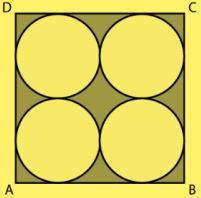Area of four plates = 4 × 154
= 616 cm2
Area of the sheet not covered by plates = 784 - 616
= 168 cm2

11. Floor of a room is of dimensions 5 m × 4 m and it is covered with circular tiles of diameters 50 cm each as shown in Fig. Find the area of floor that remains uncovered with tiles. (Use π = 3.14).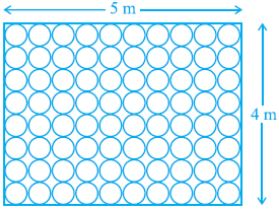Solution

Length = 5 m
Area = l × b
= 20 m2
Diameter of each circular tile = 50 cm
= 1/4 m
Now,
Area of circular tile ;
= πr2
= 3.14 × (1/4)2
= 3.14/6
Area of 80 circular tiles  = 80 × (3.14/16) = 1.57 m2
Area which remain uncovered Area of floor - Area of 80 circular tiles
= 20 - 15.7
= 4.3 m2

12. All the vertices of a rhombus lie on a circle. Find the area of the rhombus, if area of the circle is 1256 cm2 . (Use π = 3.14).

Solution

Let the radius of the circle be r.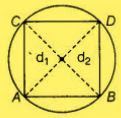Area of circle = 1256 cm2
πr2 = 1256
⇒ r = 20 cm
So, Diameter = 40 cm
As, all the vertices of a rhombus lie on a circle that means each diagonal of a rhombus must pass through the center of a circle that is why both diagonals are equal and same as the diameter of the given circle.
Let d1 and d2 be the  diagonals of rhombus,
And, Diameter of circle = 40 cm
Area of rhombus = (1/2) × d1 × d2
= (1/2) × 40 × 40
= 800 cm2

13. An archery target has three regions formed by three concentric circles as shown in Fig.. If the diameters of the concentric circles are in the ratio 1: 2:3, then find the ratio of the areas of three regions.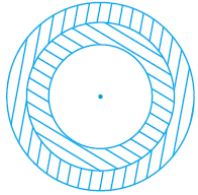Solution
Let the diameters of concentric circles be k, 2k and 3k.
Radius of concentric circle are  = k/2, k, 3k/2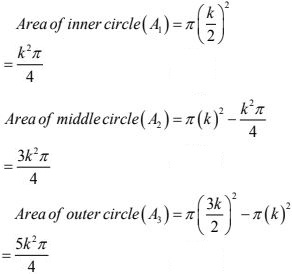Required ratio = A1 : A2 : A3
= 1 : 3 : 5

14. The length of the minute hand of a clock is 5 cm. Find the area swept by the minute hand during the time period 6 : 05 a m and 6 : 40 a m.
Solution
We know that in 60 minute hand revolve = 360°
In 1 min = 360/60 In (6 : 05 am to 6 :40 am ) = 35 minutes,
= 360/60 × 35
= 210
Length of minute hand (r) = 5 cm
Area of sector AOBA with angle O = (πr2 /360) × ∠O
(π52 /360) × 210
= 275/6
= 45(5/6) cm2

15. Area of a sector of central angle 200° of  a circle is 770 cm2 . Find the length of the corresponding arc of this sector.
Solution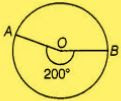Let the radius of the sector AOBA be r.
Central Angle =200°
Area of sector =770 cm2
Area of sector = (πr2 /360) × θ
⇒ 770 = (πr2 /360) × 200
⇒ r = 21 cm
Length of corresponding arc of this sector = Central angle × Radius
= 200 × 21 × π/180
= 220/3
= 77(1/3) cm

16. The central angles of two sectors of circles of radii 7 cm and 21 cm are respectively 120° and 40°. Find the areas of the two sectors as well as the lengths of the corresponding arcs. What do you observe?
Solution
Let the lengths of the corresponding arc be l1 and l2 .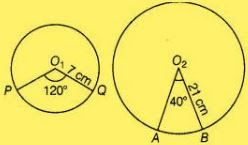And,
Central angle of sector PO1QP=120°
Central angle of sector AO2BA=40°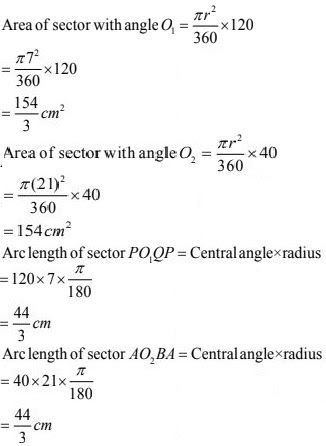Hence, we observe that arc lengths of two sectors of two different circles may be equal but their area need not be equal.

17. Find the area of the shaded region given in Fig.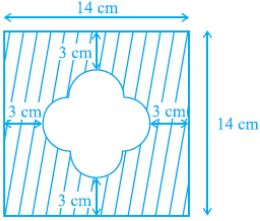Solution
Construction :
Join JK, KL, LM and MJ,
There are four equally semi - circles and LMJK formed a square.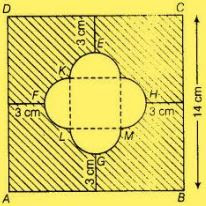FH = 14 - (3 + 3)
= 8 cm
Side of square = 4 cm
Radius of semicircle = 2 cm
Now,
Area of square JKLM = 42 = 16 cm2
Area of semicircle HJM = πr2/2
= π22/2 = 2π
= 6.28 cm2
Area of four semicircle = 4 × 6.28
= 25.12 cm2
Now,
Area of square ABCD = 142
= 196 cm2
Area of shaded region = area of square ABCD - (Area of  4 semicircle + area of square JKLM)
= 196 - (8π + 16)
= 180 - 8π  cm2

18. Find the number of revolutions made by a circular wheel of area 1.54 m2 in rolling a distance of 176 m.
Solution
Let us take the number of revolutions made by a circular wheel be n and the radius of circular wheel be r.
Area of circular wheel = 1.54 m2
πr2 = 1.54
⇒ (22/7)r2 = 1.54
⇒ r = 0.7 m
Distance travelled by circular wheel in one revolution = Circumference of circular wheel
= 2πr
= 2 × (22/7)× 0.7
= 4.4 m
Distance travelled by circular wheel = 176 m
Number of revolutions  = (Total distance)/(Distance of revolution)
= 176/4.4
= 40

19. Find the difference of the areas of two segment of a circle formed by a chord of length 5 cm subtending an angle of 90° at the centre.
Solution
Let the radius of the circle be r.
OA = OB = r cm
We have,
AB = 5 cm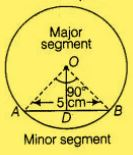Central angle of AOBA = 90°
In Δ AOB, AB2 = OA2 + OB2
52 = r2 + r2
⇒ r = 5/√2 cm
Also, AD = DB = 5/2 cm
= [5/√2)2 - [5/2]2
= 5/2 cm
Area of isosceles ΔAOB = (1/2) × base × height
= (1/2) × 5× 52
= 25/4 cm2
Area of sector AOBA = (πr2 /360) × θ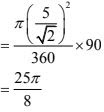Area of minor segment = Area of sector AOBA - Area of isosceles ΔAOB
= [25π/8 - 25/4]
Now, Area o circle = πr2
= π(5/√2)2
= 25π/2
Area of major segment = Area of circle  - Area of minor segment
= (25π/2) - [25π/8 - 25/4]
= (75π/8 + 25/4)
Now, Difference  = Area of major segment - Area of minor segment
= (75π/8 + 25/4) - (25π/8 - 254)
= 25π/4 + 25/4  cm2

20. Find the difference of the areas of a sector of angle 120° and its corresponding major sector of a circle of radius 21 cm.
Solution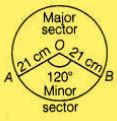Radius of the circle (r) = 21 cm and
Central angle of the sector AOBA(θ) = 120°
Area of circle = πr2
= (22/7) × (21)2
= 1386 cm2
Area of minor sector (OABA) = (πr2 /360) × θ
= (22/7) × [(21)2/360] × 120°
= 462 cm2
Area of major sector = Area of circle  - Area of min or sector
= 1386 - 432
= 924 cm2
Difference of area of major and min or sector,
= 924 - 462
= 462 cm2